U.S. Department of Transportation
1200 New Jersey Avenue, SE
Washington, DC 20590
202-366-4000

Federal Highway Administration Research and Technology
Coordinating, Developing, and Delivering Highway Transportation Innovations

 REPORT This report is an archived publication and may contain dated technical, contact, and link information
 Publication Number:  FHWA-HRT-12-031    Date:  August 2012
 Publication Number: FHWA-HRT-12-031 Date: August 2012

# User’s Guide: Estimation of Key PCC, Base, Subbase, and Pavement Engineering Properties From Routine Tests and Physical Characteristics

PDF files can be viewed with the Acrobat® Reader®

## PCC Flexural Strength Models

### Validation of Existing Models

Previous models correlating flexural strength to compressive strength have generally used a power model of the following form: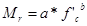Figure 38. Equation. Mr.

Where:

a = 7.5 to 11.7 for b = 0.5.
a = 2 to 2.7 for b = 0.67.

Table 11 shows a summary of the models developed. The regressed constants, a and b, were found to be within the range of values reported by the other studies. This validation not only provides feasible models, but it also confirms that the data used reasonably represent the broad range considered in the various studies. The correlations are presented in figure 39 and figure 40 for the power models with exponents of 0.5 and 0.67, respectively.

Table 11. Power models developed for flexural strength prediction using LTPP data for validation.

 Model a b R2 N10.3022 0.50 0.446 185 2.4277 0.67 0.449 185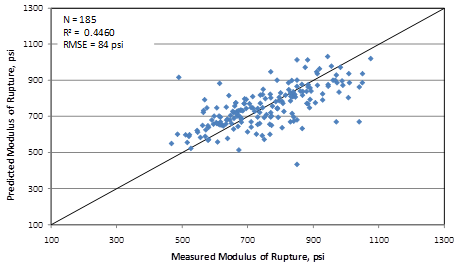Figure 39. Graph. Predicted versus measured for validating 0.5 power flexural strength model.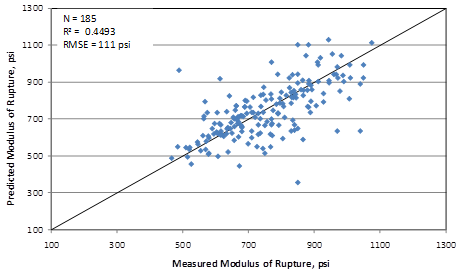Figure 40. Graph. Predicted versus measured for validating 0.667 power flexural strength model.

### Flexural Strength Model 1: Flexural Strength Based on Compressive Strength

This model provides the best correlation between compressive strength and flexural strength with the LTPP data. The model form utilizes the power equation. This model will be most useful for cases when the compressive strength of the PCC has been determined through a routine cylinder break. This model can be expressed as follows:

Figure 41. Equation. Prediction model 6 for MR.

Where:

MR = Flexural strength, psi.
f'c = Compressive strength determined at the same age, psi.

The regression statistics for this model are presented in table 12. The model was developed using 185 data points, and the prediction has an R2 value of 45.2 percent and an RMSE of 69 psi. Table 13 provides details of the range of data used to develop the model.

Table 12. Regression statistics for flexural strength model based on compressive strength.

 Parameter Estimate Standard Error Approximate 95 Percent Confidence Limits a 22.7741 6.6362 9.6807 to 35.8674 b 0.4082 0.0338 0.3416 to 0.4748

Table 13. Range of data used for flexural strength model based on compressive strength.

 Parameter Minimum Maximum Average Compressive strength 1,770 10,032 5,431 Flexural strength 467 1,075 754

Figure 42 and figure 43 show the predicted versus measured plot and the residual plot, respectively.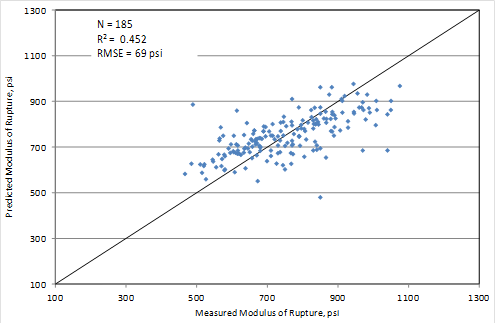Figure 42. Graph. Predicted versus measured values for flexural strength model based on compressive strength.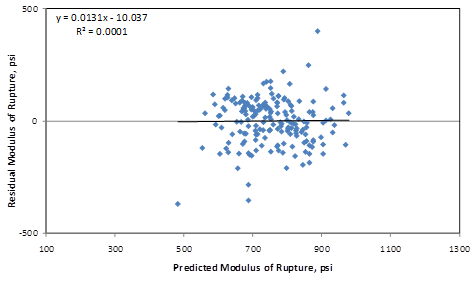Figure 43. Graph. Residual errors for flexural strength model based on compressive strength.

Figure 44 shows a comparison of the power models used to validate the data and develop a new correlation. Note that the three power models (the new equation developed for this study as well as the validation models) provide close estimates (within 50 psi) in the 4,500- to 5,500-psi compressive strength range.

The American Concrete Institute (ACI) and Portland Cement Association (PCA) models are plotted for comparison. Also plotted are the raw data that were used in the model. Clearly, the ACI equation is conservative for this data. It has also been found to give a conservative estimate for several large datasets that have been used in flexural strength model prediction. Conversely, the PCA model fits the LTPP data more closely. The reasons for this lack of fit of the current data with the previous models may be too many to fully explain. The data used in models from prior studies often came from mixes batched under controlled laboratory experiments and were typical of paving and structural concrete. The mixes used in the current model developed from LTPP data relies on only mixes proportioned for typical paving operations. Furthermore, the LTPP data used are from many projects widely dispersed around the United States. This in itself makes the models more robust than any previous data used to make similar correlations.

The spread in the raw data about the prediction model in figure 44 clearly indicates that there are factors other than compressive strength that influence the flexural strength of PCC. Among the various factors influencing flexural strength are the mix design parameters and age of the concrete. These variables are considered in the other models developed in this study.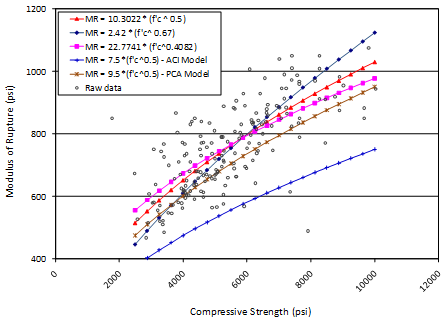Figure 44. Graph. Comparison of flexural strength models based on compressive strength.

### Flexural Strength Model 2: Flexural Strength Based on Age, Unit Weight, and w/c Ratio

Flexural strength model 2 provides a correlation between flexural strength and mix design parameters, specifically the unit weight and w/c ratio. Age is also a parameter in this model, which helps reduce some of the variability seen in the prediction relative to the predictions shown in figure 44. This model will be most useful for cases when the compressive strength of PCC is not determined but mix design information is available. Also, the user has the option of predicting the 28-day strength value for design or estimating the strength at traffic opening time.

This model can be expressed as follows: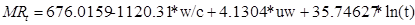Figure 45. Equation. Prediction model 7 for MRt.

Where:

MRt = Flexural strength at age t years, psi.
w/c = w/c ratio.
uw = Unit weight, lb/ft3.
t = Pavement age, years.

The regression statistics for this model are presented in table 14. The model was developed using 62 data points, and the prediction has an R2 value of 61.11 percent and an RMSE of 91 psi. Table 15 provides details of the range of data used to develop the model.

Table 14. Regression statistics for flexural strength model based on age, unit weight, and w/c ratio.

 Variable DF Estimate Standard Error t-Value Pr > |t| VIF Intercept 1 676.0159 277.7887 2.43 0.0181 0 w/c 1 -1,120.31 141.3573 -7.93 < 0.0001 1.00591 Unit weight 1 4.1304 1.88934 2.19 0.0329 1.00311 Ln(age) 1 35.74627 8.78516 4.07 0.0001 1.00619

Table 15. Range of data used for flexural strength model based on age, unit weight, and w/c ratio.

 Parameter Minimum Maximum Average w/c ratio 0.27 0.58 0.40 Unit weight 124 151 142 Pavement age 0.0384 1.0000 0.3169 Flexural strength 467 978 742

Figure 46 and figure 47 show the predicted versus measured plot and the residual plot, respectively.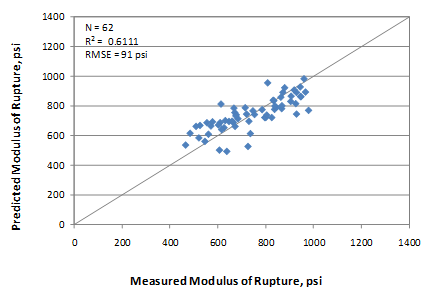Figure 46. Graph. Predicted versus measured values for flexural strength model based on age, unit weight, and w/c ratio.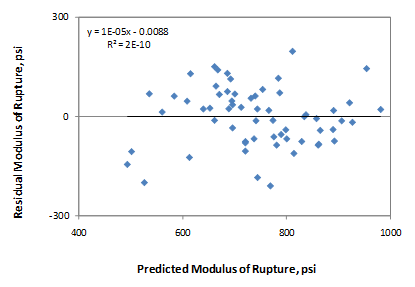Figure 47. Graph. Residual errors for flexural strength model based on age, unit weight, and w/c ratio.

### Flexural Strength Model 3: Flexural Strength Based on Age, Unit Weight, and CMC

The model is expressed as follows: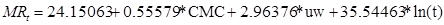Figure 48. Equation. Prediction model 8 for MRt.

Where:

MRt = Flexural strength at age t years, psi.
CMC = Cementitious materials content, lb/yd3.
uw = Unit weight, lb/ft3.
t = Pavement age, years.

The regression statistics for this model are presented in table 16. The model was developed using 62 data points, and the prediction has an R2 value of 70.23 percent and RMSE of 80 psi. Table 17 provides details of the range of data used to develop the model.

Table 16. Regression statistics for flexural strength model based on age, unit weight, and CMC.

 Variable DF Estimate Standard Error t-Value Pr > |t| VIF Intercept 1 24.15063 236.7606 0.1 0.9191 0 CMC 1 0.55579 0.05563 9.99 < 0.0001 1.01522 Unit weight 1 2.96376 1.66087 1.78 0.0796 1.01253 Ln(age) 1 35.54463 7.68504 4.63 < 0.0001 1.00573

Table 17. Range of data used for flexural strength model based on age, unit weight, and CMC.

 Parameter Minimum Maximum Average CMC 388 936 668 Unit weight 124 151 142 Pavement age 0.0384 1.0000 0.3169 Flexural strength 467 978 742

Figure 49 and figure 50 show the predicted versus measured plot and the residual plot, respectively.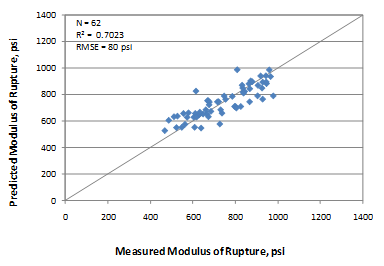Figure 49. Graph. Predicted versus measured values for flexural strength model based on age, unit weight, and CMC.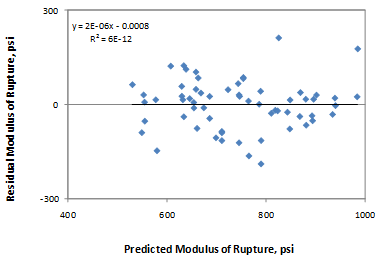Figure 50. Graph. Residual errors for flexural strength model based on age, unit weight, and CMC.

Figure 51 through figure 54 present the sensitivity of the mix design-based flexural strength models to CMC, w/c ratio, unit weight, and age. Figure 51 and figure 52 show that prediction models 7 and 8 do not show any sensitivity to CMC and w/c ratio (see figure 45 and figure 48). For typical values of these parameters, the flexural strength prediction from these two models could show a difference of about 200 psi for extreme values of w/c ratios. However, within a typical range of 0.35 to 0.45, the flexural strength prediction is within 50 psi. Similar trends are evident for the w/c ratio parameter. Therefore, if all details about a mix design are available, it is highly recommended that both models be used to predict flexural strength so that the user has a fair estimate of the flexural strength range. Figure 53 shows that the predictions are close from both models. Likewise, figure 54, which is more or less a flexural strength gain model for a typical mix design, shows very close predictions from both models.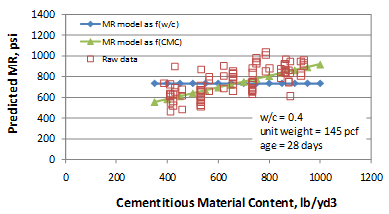Figure 51. Graph. Sensitivity of flexural strength predictions to CMC.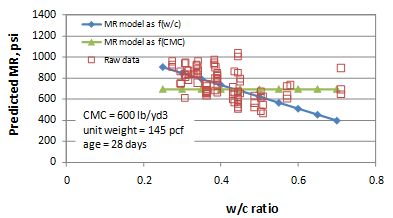Figure 52. Graph. Sensitivity of flexural strength predictions to w/c ratio.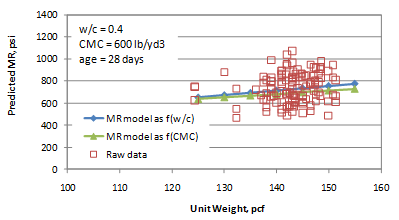Figure 53. Graph. Sensitivity of flexural strength predictions to unit weight.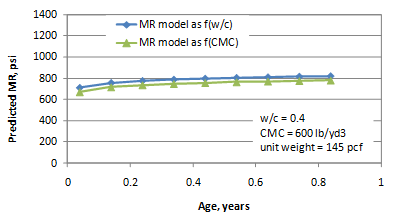Figure 54. Graph. Sensitivity of flexural strength predictions to age.

Federal Highway Administration | 1200 New Jersey Avenue, SE | Washington, DC 20590 | 202-366-4000
Turner-Fairbank Highway Research Center | 6300 Georgetown Pike | McLean, VA | 22101